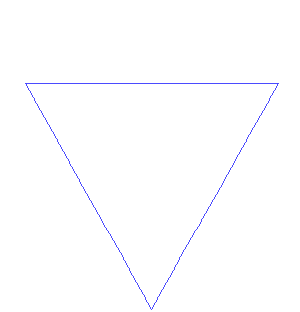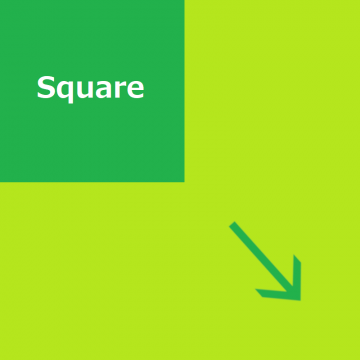# Are All Fractals Complex Systems?

2000-05-28

Although almost all books concerning complex systems mention chaos and fractals, I suspect that not all fractals are complex systems.I have already explained chaos and complex systems. So, first I must explain what fractals are.

## 1. What is a fractal?

Mandelbrot defined a fractal as a shape made of parts similar to the whole in some way. For example, if you reiterate the process of dividing a line segment into three equal parts and doubling the length of the second line segment forming two sides of an equilateral triangle, you will get the Koch curve.Koch curve. The first 7 orders of the Koch curve discovered by Helge von Koch . Source: António Miguel de Campos. “7 first steps of the building of the von Koch curve in animated gif.” 15 May 2007. Licensed under CC-0.

The Koch curve consists of four self-similar parts 1/4 the size of the whole. Mandelbrot introduced a mathematical parameter called fractal dimension.Fractal Dimension. If you double each side of a square on a 2-dimensional plane, you will get 4 (22) squares of the original size.

If you double each side of a cube in 3-dimensional space, you will get 8 (23) cubes of the original size. Hereby we can define the fractal dimension of a figure as d, whose parts are n raised to the d-th power when magnified n times. The fractal dimension of the Koch curve denotes the power to which 3 must be raised to produce 4:

3d = 4

The dimension is thus log4/log3, or roughly 1.26.

The term fractal derived from a Latin word fractus meaning “fragmented" or “broken". That’s because fractal dimension is, unlike Euclidean dimension, generally expressed by a non-integer, that is to say, by a fraction rather than by a whole number.

If a whole is composed of two or more self-similar parts, it is logically impossible for parts to project the whole completely on themselves. If the whole is at the level n+1 and the parts are at the level n with the difference between them fixed, parts cannot catch up with the whole. However, when n is magnified up to infinity, the difference between whole and part will disappear, and the length of the Koch curve, n-th power of 4/3 is also magnified up to infinity.

Some might not consider self-similarity to be a necessary and sufficient condition for fractals.

First, is self-similarity a sufficient condition? When you record white noises and hear them at a half or double speed, they sound just the same as at an original speed. White noises have self-similarity in this sense but they are not fractals because it is impossible to argue the fractal dimension of white noises.

Second, is self-similarity a necessary condition? If self-similarity is taken strictly, most of nature turns out to have few fractal structures. So, some scientists insist that fractals do not have to be self-similar.

Anyway, if you are not a specialist, you can regard fractals as self-similar patterns without committing serious mistakes.

## 2. How are fractals related to chaos?

This much will do for explaining fractals. Now I can return to the main question. Are all fractals complex systems? How are fractals related to chaos?

Chaos and fractals have certainly something in common. The following are important common characteristics:

1. You cannot reduce the whole to parts but to rules.
2. They are brought about by self-referential repetitive application of simple rules.

Let’s examine each case.

### 2.1. Modification of reductionism

Modern scientists have analyzed objects by reducing an unintelligible whole to intelligible parts. But when the unintelligible whole is composed of similarly unintelligible parts, reductionism proves to be helpless. In other words, you cannot depend on differential/integral calculus. Differential calculus is based on typical reductionism that reduces a nonlinear whole into linear parts.

A fractal is an unmanageable function for modern scientists, because it can be differentiated nowhere, though it is continuous. For modern mathematicians linear algebra was fundamental and the nowhere differentiable continuous functions were treated as exceptional. In a real nature however it is linear algebra that is exceptional and most of nature has a fractal structure.

Chaos also refuses to be reduced to parts. Most of chaos including Lorenz attractor has a fractal structure. Its “strange" whole can be reduced not to parts but to rules.

This reduction to rules has some commercial advantage. Plotting the bit map of complicated wooden branches and leaves on a computer screen makes its data size too large, while applying fractal rules compresses the data size. Such fractal compression technology was also applied to color pictures of Microsoft encyclopedia “Encarta". Otherwise, so many pictures cannot have been stored in one CD-ROM.

In order to generate fractal pictures you can use not only non-random fractals like Mandelbrot sets, but also random fractals, which draw complicated patterns of fractal nature such as coastlines, trees, electric discharge, cloud and so on with varying parameters mediated.

Fractal figures are “complex" in common-sense terms but non-random fractals like Koch curve have periodicity and no orbital instability (Lyapunov spectrum). Fractals differ from chaos at this point.

### 2.2. self-referential repetitive application

Chaos is brought about by self-referential repetitive application of nonlinear functions as was written at the former issue, but I must mention exceptions. When the constant a in the logistic map

f (x) = ax (1-x)

is three or less, self-referential repetitive application of it does not bring about chaos but convergence to a single stable fixed point, or fixed periodicity.

Let’s take a non-mathematical example. A proposition

" This proposition is true. “

is self-referential and self-consistent, but

" This proposition is false. “

is false, if true, and true, if false, so it has no determinate truth-value. This is a well-known liar paradox and the Russell’s Paradox of set theory and Goedel’s incompleteness theorem succeeded this paradoxical logic found by the Ancients. Here you must note that the self-reference itself does not bring about paradox.

The self-similarity of fractals corresponds to the self-reference of chaos. Taking similarity narrowly prevents fractals from being complex systems. Taking similarity widely, you can call complex, namely unpredictable behaviors of market indices fractals, for example. In charts, you find similar wave-like patterns at one day or one month or one year long period and similar technical analysis is available on all levels. This means stock charts are random fractals. If stock charts were non-random, you could predict the future prices of stocks and be a millionaire using computer simulation.

## 3. My conclusion

My consideration 1+2 concludes that not all fractals are complex systems, just as not all self-referential repetitive application of nonlinear functions causes chaos.